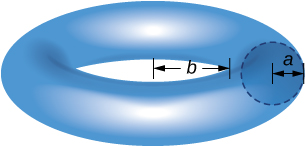## Problem Set: Moments and Centers of Mass

For the following exercises (1-6), calculate the center of mass for the collection of masses given.

1. ${m}_{1}=2$ at ${x}_{1}=1$ and ${m}_{2}=4$ at ${x}_{2}=2$

2. ${m}_{1}=1$ at ${x}_{1}=-1$ and ${m}_{2}=3$ at ${x}_{2}=2$

3. $m=3$ at $x=0,1,2,6$

4. Unit masses at $(x,y)=(1,0),(0,1),(1,1)$

5. ${m}_{1}=1$ at $(1,0)$ and ${m}_{2}=4$ at $(0,1)$

6. ${m}_{1}=1$ at $(1,0)$ and ${m}_{2}=3$ at $(2,2)$

For the following exercises (7-16), compute the center of mass $\overline{x}.$

7. $\rho =1$ for $x\in (-1,3)$

8. $\rho ={x}^{2}$ for $x\in (0,L)$

9. $\rho =1$ for $x\in (0,1)$ and $\rho =2$ for $x\in (1,2)$

10. $\rho = \sin x$ for $x\in (0,\pi )$

11. $\rho = \cos x$ for $x\in (0,\frac{\pi }{2})$

12. $\rho ={e}^{x}$ for $x\in (0,2)$

13. $\rho ={x}^{3}+x{e}^{\text{−}x}$ for $x\in (0,1)$

14. $\rho =x \sin x$ for $x\in (0,\pi )$

15. $\rho =\sqrt{x}$ for $x\in (1,4)$

16. $\rho =\text{ln}x$ for $x\in (1,e)$

For the following exercises (17-), compute the center of mass $(\overline{x},\overline{y}).$ Use symmetry to help locate the center of mass whenever possible.

17. $\rho =7$ in the square $0\le x\le 1,$ $0\le y\le 1$

18. $\rho =3$ in the triangle with vertices $(0,0),$ $(a,0),$ and $(0,b)$

19. $\rho =2$ for the region bounded by $y= \cos (x),$ $y=\text{−} \cos (x),$ $x=-\frac{\pi }{2},$ and $x=\frac{\pi }{2}$

For the following exercises, use a calculator to draw the region, then compute the center of mass $(\overline{x},\overline{y}).$ Use symmetry to help locate the center of mass whenever possible.

20. [T] The region bounded by $y= \cos (2x),$ $x=-\frac{\pi }{4},$ and $x=\frac{\pi }{4}$

21. [T] The region between $y=2{x}^{2},$ $y=0,$ $x=0,$ and $x=1$

22. [T] The region between $y=\frac{5}{4}{x}^{2}$ and $y=5$

23. [T] Region between $y=\sqrt{x},$ $y=\text{ln}(x),$ $x=1,$ and $x=4$

24. [T] The region bounded by $y=0,$ $\frac{{x}^{2}}{4}+\frac{{y}^{2}}{9}=1$

25. [T] The region bounded by $y=0,$ $x=0,$ and $\frac{{x}^{2}}{4}+\frac{{y}^{2}}{9}=1$

26. [T] The region bounded by $y={x}^{2}$ and $y={x}^{4}$ in the first quadrant

For the following exercises, use the theorem of Pappus to determine the volume of the shape.

27. Rotating $y=mx$ around the $x$-axis between $x=0$ and $x=1$

28. Rotating $y=mx$ around the $y$-axis between $x=0$ and $x=1$

29. A general cone created by rotating a triangle with vertices $(0,0),$ $(a,0),$ and $(0,b)$ around the $y$-axis. Does your answer agree with the volume of a cone?

30. A general cylinder created by rotating a rectangle with vertices $(0,0),$ $(a,0),(0,b),$ and $(a,b)$ around the $y$-axis. Does your answer agree with the volume of a cylinder?

31. A sphere created by rotating a semicircle with radius $a$ around the $y$-axis. Does your answer agree with the volume of a sphere?

For the following exercises, use a calculator to draw the region enclosed by the curve. Find the area $M$ and the centroid $(\overline{x},\overline{y})$ for the given shapes. Use symmetry to help locate the center of mass whenever possible.

32. [T] Quarter-circle: $y=\sqrt{1-{x}^{2}},$ $y=0,$ and $x=0$

33. [T] Triangle: $y=x,$ $y=2-x,$ and $y=0$

34. [T] Lens: $y={x}^{2}$ and $y=x$

35. [T] Ring: ${y}^{2}+{x}^{2}=1$ and ${y}^{2}+{x}^{2}=4$

36. [T] Half-ring: ${y}^{2}+{x}^{2}=1,$ ${y}^{2}+{x}^{2}=4,$ and $y=0$

37. Find the generalized center of mass in the sliver between $y={x}^{a}$ and $y={x}^{b}$ with $a>b.$ Then, use the Pappus theorem to find the volume of the solid generated when revolving around the $y$-axis.

38. Find the generalized center of mass between $y={a}^{2}-{x}^{2},$ $x=0,$ and $y=0.$ Then, use the Pappus theorem to find the volume of the solid generated when revolving around the $y$-axis.

39. Find the generalized center of mass between $y=b \sin (ax),$ $x=0,$ and $x=\frac{\pi }{a}.$ Then, use the Pappus theorem to find the volume of the solid generated when revolving around the $y$-axis.

40. Use the theorem of Pappus to find the volume of a torus (pictured here). Assume that a disk of radius $a$ is positioned with the left end of the circle at $x=b,$ $b>0,$ and is rotated around the $y$-axis.41. Find the center of mass $(\overline{x},\overline{y})$ for a thin wire along the semicircle $y=\sqrt{1-{x}^{2}}$ with unit mass.

(Hint: Use the theorem of Pappus.)